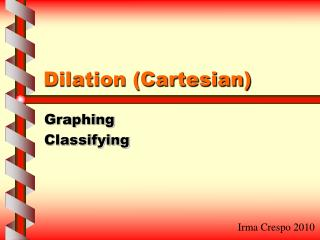DownloadDownload PresentationDilation (Cartesian)

# Dilation (Cartesian)

Download Presentation## Dilation (Cartesian)

- - - - - - - - - - - - - - - - - - - - - - - - - - - E N D - - - - - - - - - - - - - - - - - - - - - - - - - - -
##### Presentation Transcript

1. Dilation (Cartesian) Graphing Classifying Irma Crespo 2010

2. original copy 36 48 Raymond’s Warm Up • A man copies a picture to a certain size but does not know how big he made it. Get the scale factor of the picture to find how big the picture was made. • 5 • b) 8 • 6 • 7 6 8 Author: Raymond Morrow April 2010

3. mom Devin’s Warm Up • There are two geckos: a mom and a baby. It takes two years for a baby gecko to grow the size of its mom. If the baby gecko is half the size of its 10 inches by 2 inches mom, what is the size of the baby? 10 inches • 5 in by 2 in • b) 20 in by 4 in • 5 in by 4 in • 5 in by 1 in 2 inches baby Author: Devin Seamans April 2010

4. Dilation is enlarging or reducing a figure by a scale factor. Dilation

5. C B A D Draw a Dilation Scale Factor: 2 Center Point: A C’ B’ D’ Enlargement of Polygon ABCD

6. Ordered Pairs in Dilation Scale Factor: 2 ABCD AB’C’D’ (4,4) D(2,0) D(2 *2,0 *2) D’(4,0) (0,2) C(2,2) C(2 *2,2 *2) C’(4,4) (2,2) B(0,1) (0,1) B(0 *2,1 *2) B’(0,2) A(0,0) A(0*2,0*2) A(0,0) (2,0) (0,0) (4,0)

7. On the Graphing Board • Plot triangle JKL with the following ordered pairs (x, y): • J(3,8) • K(10,6) • L(8,2) • Plot triangle J’K’L’ with a scale factor of ½ . J(3,8) J(3* ½,8* ½) J’(1.5,4) K(10,6) K(10* ½,6* ½) K’(5,3) L(8,2) L(8* ½,2* ½) L’(4,1)

8. On the Graphing Board • Plot triangle JKL with the following ordered pairs (x, y): • J(3,8) • K(10,6) • L(8,2) • Plot triangle J’K’L’ with a scale factor of 2. J(3,8) J(3* 2,8* 2) J’(6,16) K(10,6) K(10* 2,6* 2) K’(20,12) L(8,2) L(8* 2,2*2) L’(16,4)

9. Z’ X’ V’ Z X W’ V W Find and Classify a Scale Factor COPY • Quadrilateral V’Z’X’W’ is a dilation of quadrilateral VZXW. ORIGINAL

10. Z’ X’ V’ Z X W’ V W Find the Scale Factor • Pick corresponding points (ordered pairs). • Make a ratio of either the Xs or the Ys following: coordinate point of copy coordinate point of original V(-2,2) and V’(-5,5) -5 -2 5 2 V’ V OR

11. Z’ X’ V’ Z X W’ V W Classify the Scale Factor 5 2 • Scale factor is • Is it less than 1? NO • So, it is not a reduction. • Is it greater than 1? YES • It is an enlargement.

12. B B’ A’ C’ A C Your TurnFind and Classify the Scale Factor (OHP) • Triangle A’B’C’ is a dilation of ABC.

13. Dilation is enlarging or reducing a figure by a scale factor. • To graph a dilation given ordered pairs and scale factor: • *multiply the scale factor to each coordinate point of each ordered pair • *plot the scaled ordered pairs • *connect the points with line segment • To find the scale factor, pick corresponding ordered pairs and create the ratio of • A dilation is an enlargement if the scale factor is greater than 1. • A dilation is a reduction if the scale factor is less than 1. coordinate point of copy coordinate point of original Summary

14. Exit Slip • Plot line segment XY with the following ordered pairs: • X(-4,2) • Y(2,6) • Plot line segment X’Y’ with a scale factor of ½. • . • Classify if the dilation is enlargement or reduction. EXPLAIN! • .

15. Complete the practice worksheet. Skills Practice: Lesson 4-8, Page 54 Work with a partner or on your own. Submit completed worksheet for grading. Solutions are discussed the next day. Practice Worksheet

16. Main Resources • Math Connects: Concepts, Skills, and Problem Solving; Teacher Edition; Course 3, Volume 1 • Columbus:McGraw-Hill, 2009. • PowerPoint created by Irma Crespo. University of Michigan-Dearborn, School of Education. Winter 2010. Lesson plan put together by Irma Crespo.